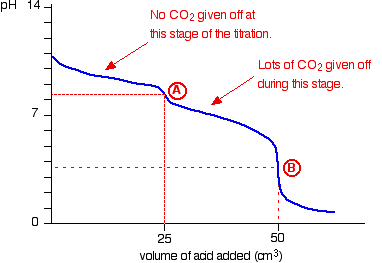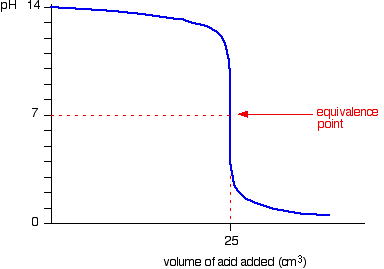# Question #dfbe7

Sep 26, 2016

The solution contains 0.025 mol of NaOH or 1 g.

#### Explanation:

The equations for the two neutralisation reactions are :

$\textsf{N {a}_{2} C {O}_{3 \left(a q\right)} + 2 H C {l}_{\left(a q\right)} \rightarrow 2 N a C {l}_{\left(a q\right)} + C {O}_{2 \left(g\right)} + {H}_{2} {O}_{\left(l\right)}}$

and

$\textsf{N a O {H}_{\left(a q\right)} + H C {l}_{\left(a q\right)} \rightarrow N a C {l}_{\left(a q\right)} + {H}_{2} {O}_{\left(l\right)}}$

The first reaction occurs in 2 stages during the titration.

The 1st proton is attached at about pH = 8.3:

$\textsf{C {O}_{3}^{2 -} + {H}^{+} \rightarrow H C {O}_{3}^{-} \text{ } \textcolor{red}{\left(1\right)}}$

So at this stage of the titration no $\textsf{C {O}_{2}}$ is observed.

At about pH = 3.7 the 2nd proton is attached:

$\textsf{H C {O}_{3}^{-} + {H}^{+} \rightarrow {H}_{2} C {O}_{3} \rightarrow {H}_{2} O + C {O}_{2} \text{ } \textcolor{red}{\left(2\right)}}$

The production of $\textsf{C {O}_{2}}$ is now observed.

The change in pH as the titration progresses can be measured with a pH meter and plotted on a graph:Phenolphthalein is an indicator which changes colour between the pH range of 8.3 - 10

The point where the indicator changes colour is called the "end - point". You want this to coincide with the "equivalence - point" when the reactants are in the amounts as specified in the equation.

This is the case for reaction $\textcolor{red}{\left(1\right)}$ at point A.

Point A on the graph lies within this range so the phenolphthalein will turn from pink to colourless at this point when 300 ml* of 0.1M HCl is added.

Normality is an obsolete quantity so I will use Molarity, which happens to be the same in this case.

If you look at the pH curve for neutralising NaOH solution with dilute HCl you can see that there is a rapid pH change between pH 11.3 and 2.7This means that phenolphthalein can also be used in this titration to give the end - point.

We can conclude then that $\textsf{C {O}_{3}^{2 -}}$ ions are being protonated and $\textsf{O {H}^{-}}$ are being neutralised at point A.

$\textsf{n H C l = c \times v = 0.1 \times \frac{300}{1000} = 0.03}$

$\therefore$$\textsf{n \left(C {O}_{3}^{2 -} + O {H}^{-}\right) = 0.03 \text{ } \textcolor{red}{\left(3\right)}}$

Now methyl orange indicator is added and this changes colour when a further 25 ml of 0.2M HCl is added.

Methyl orange changes colour within the pH range 3.1 - 4.4.

This will change at point B on the graph and indicates the equivalence point of reaction $\textcolor{red}{\left(2\right)}$.

$\textsf{{n}_{{H}^{+}} = c \times v = 0.2 \times \frac{25}{1000} = 0.005}$

From the 1:1 stoichiometry of reaction $\textcolor{red}{\left(2\right)}$ we can say that:

$\textsf{{n}_{H C {O}_{3}^{-}} = 0.005}$

This means that the original number of moles of $\textsf{C {O}_{3}^{2 -} = 0.005}$.

So from $\textsf{\textcolor{red}{\left(3\right)}}$ we get:

$\textsf{0.005 + O {H}^{-} = 0.03}$

$\therefore$$\textsf{n O {H}^{-} = 0.03 - 0.005 = 0.025}$

To get the mass of NaOH:

$\textsf{m = n \times {M}_{r} = 0.025 \times 40.0 = 1.0 \textcolor{w h i t e}{x} g}$

• 300 ml is a ridiculously large volume for a titration. 1 M HCl would bring the titre down to 30 ml.